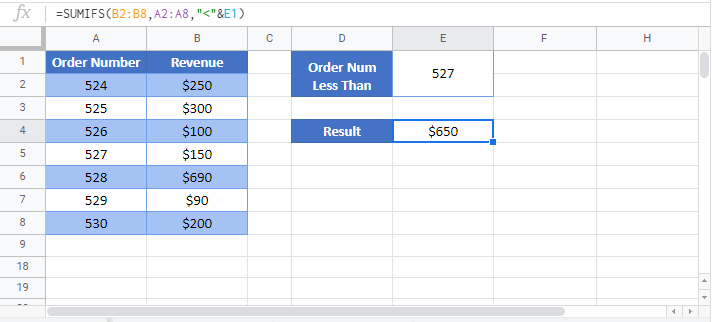# Sum If Less Than or Equal To – Excel & Google Sheets

This tutorial will demonstrate how to use the SUMIFS Function to sum rows with data less than (or equal to) a specific value in Excel and Google Sheets.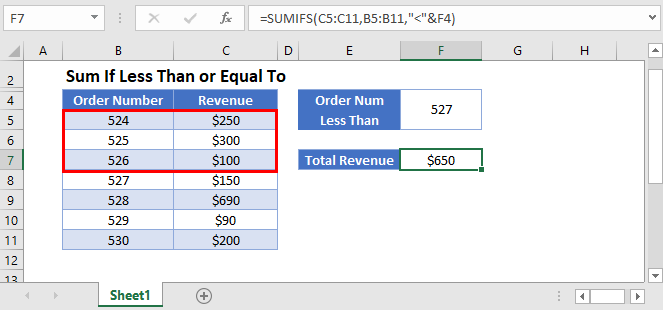## Sum if Less Than 0

The SUMIFS Function sums data rows that meet certain criteria. Its syntax is: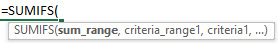This example will sum all Scores that are less than zero.

``=SUMIFS(C3:C9,C3:C9,"<0")``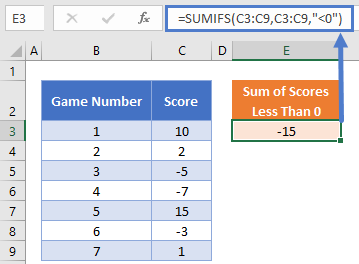We include the criteria “<0” to sum all negative Scores. In this example, the sum_range and criteria_range are the same.

## Sum if Less Than

This example sums the Revenue for all Order Numbers less than 527.

``=SUMIFS(C3:C9,B3:B9,"<527")``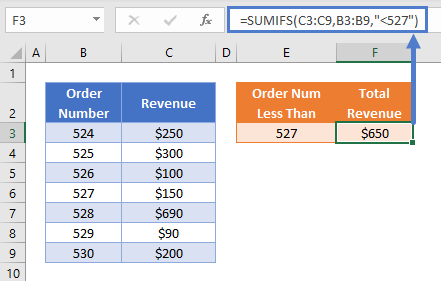### Sum if Less Than or Equal to

Instead if you’d like to include all Order Numbers less than or equal to, modify the criteria to be “<=527”:

``=SUMIFS(C3:C9,B3:B9,"<=527")``

### Sum if Less Than – Cell References

Usually, it is bad practice to hard-code values into formulas. Instead, it is more flexible to use a separate cell to define the criteria’s value.

``=SUMIFS(C3:C9,B3:B9,"<"&E3)``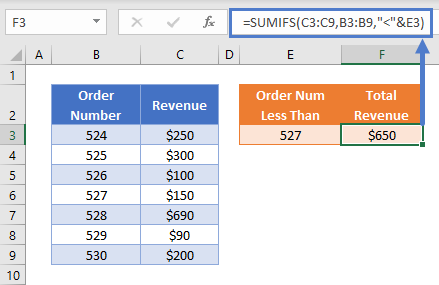Now we add the logical operator within double quotes (“”) and use & to join the operator and the criteria value:

“<“&E3

Other logical operators can be used in this formula, such as:

• Equal to (“=”&E3)
• Less than or equal to (example: “<=”&E3)
• Greater than (“>”&E3)
• Greater than or equal to ( “>=”&E3)
• Not equal to (“<>”&E3)

## Locking Cell References

To make our formulas easier to read, we’ve shown the formulas without locked cell references:

``=SUMIFS(C3:C9,B3:B9,"<"&E3)``

But these formulas will not work properly when copy and pasted elsewhere in your file. Instead, you should use locked cell references like this:

``=SUMIFS(\$C\$3:\$C\$9,\$B\$3:\$B\$9,"<"&E3)``